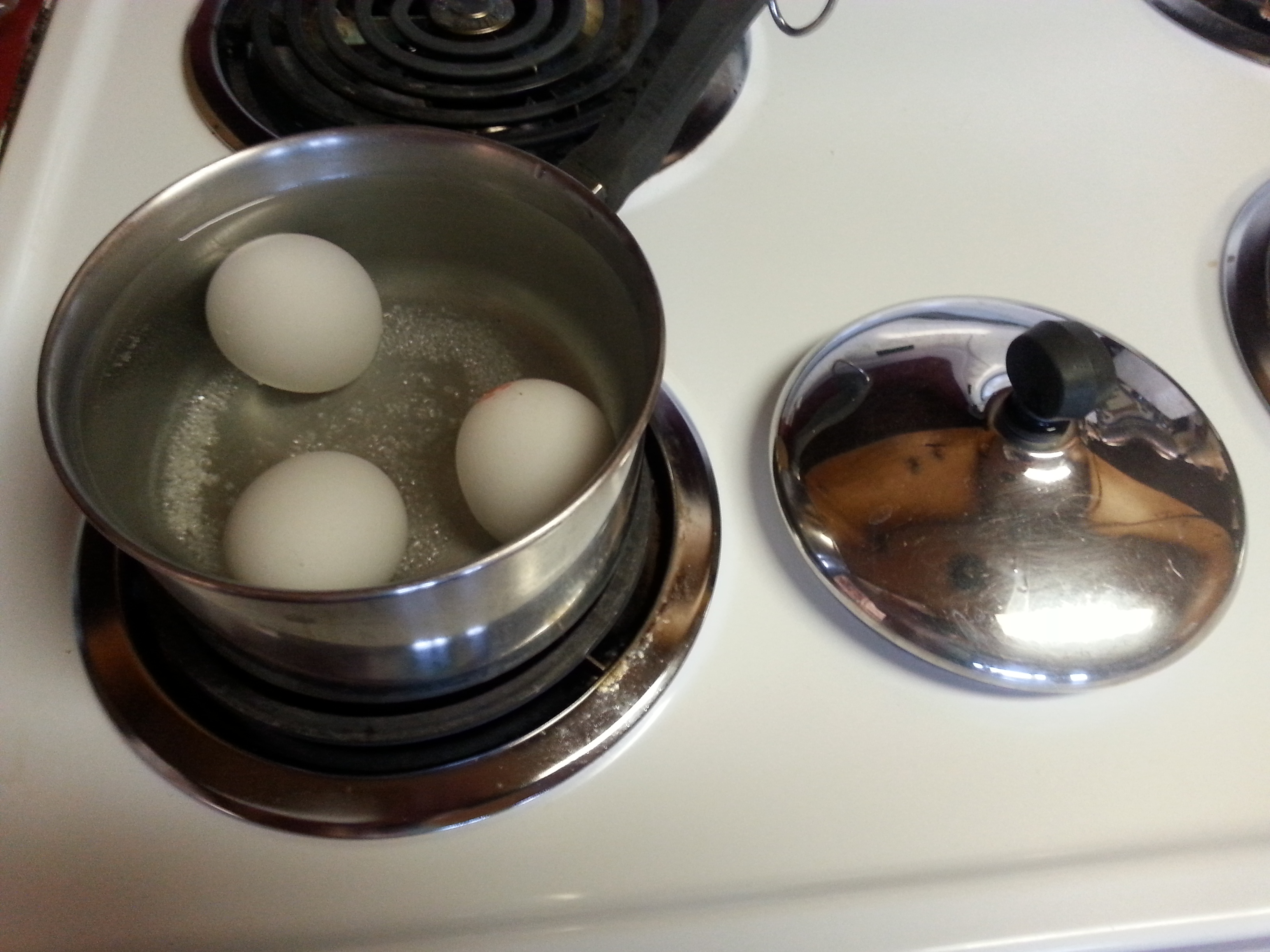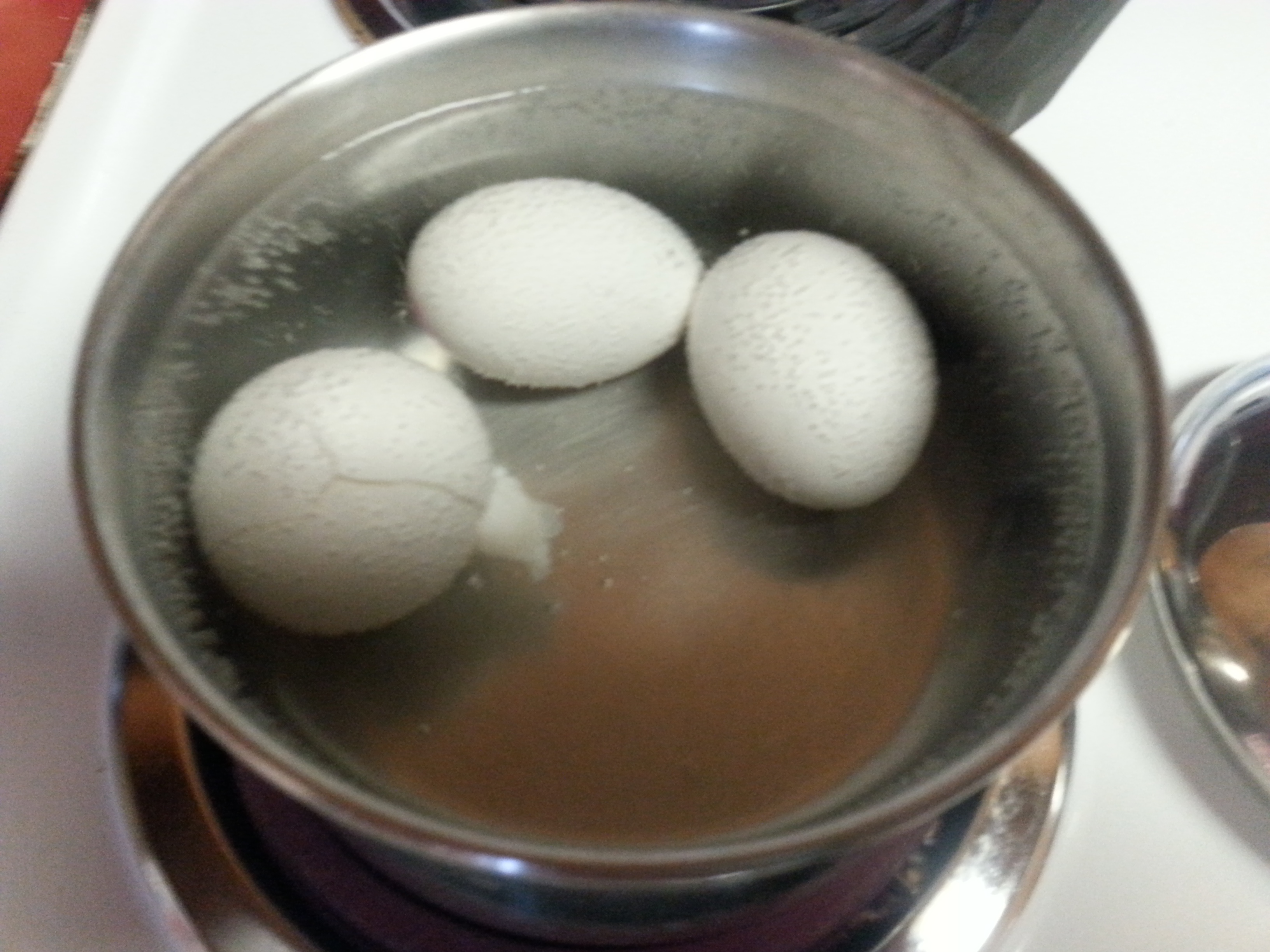## One more Javascript function

This function will format a value expressed as feet (with decimals) into feet and inches.

```// Convert feet (decimal) into feet and inches
function toString(value)
{
var abs = Math.abs(value);
// Round to nearest 0.1 inch
var rounded = Math.round(120 * abs) / 120;

var feet = Math.floor(rounded);
var inches = 12*(rounded - feet);

return (value < 0 ? "-" : "")
+ (feet != 0 ? feet + "'" : "")
+ (inches.toFixed(1) + '"');
}
```

## Perfect Hard-boiled EggsPlace eggs into salted water that covers them by at least 1/2 inch.Bring to a good boil, remove from heat and cover them. Let sit for 15 minutes. One of the eggs developed a tiny crack, and lost some white. No problemo!After they have cooked, drain the hot water and use ice and water to chill the eggs thoroughly.To peel them, crack the shell slightly all over the egg. Put them one at a time into a container with about 1 inch of water. The container should be large enough that the egg has plenty of room to move.Put the lid on and shake the container vigorously. The water will help to prevent damage to the egg and float the shell away from the egg.Here they are, after about 1 minute of work. And note that the yolks are perfectly done.## Javascript functions for numeric input and output

As a part of a calculator for model railroad stub switches, I developed a couple of javascript routines for input and output that allow more flexibility than the standard implementation.

This function converts to text for display from a numeric value, with a variable number of decimals, removing extra trailing zeroes. It also converts the (default inch) measure to millimeters based on the units global variable.

```// Smarter formatting function value, decimals
function toVariable(v, d)
{
if (units == "mm")
v = v * 25.4;
var r = v.toFixed(d).replace(/0+\$/g, '');
if (r.substring(r.length - 1) == '.')
{
r = r.substring(0, r.length - 1);
}
return r;
}
```

This function accepts text from an input box in various formats and units of measurement. The return value is always converted into inches.
Value formats:

• Decimal: a value such as 1.5
• Mixed fraction: a value such as 1 1/2
• Fraction: a value such as 3/8
• Decimal: a value such as 0.375

Measure specifiers:

• in: inches
• ft: feet
• mm: millimeter
• cm: centimeter
```// Parse a measurement in mm, cm, in or ft
// Always returns the value converted to inches
function parseMeasure(text)
{
// Mixed ex: 1 1/2 in
var re = /(\d+)\s+(\d+)\/(\d+)\s*(mm|cm|in|ft)?\.?/;
var matches = text.match(re);

var value;
var num, den;
var measure;

if (matches && matches.length > 0)
{
value = matches;
num = matches;
den = matches;
measure = (matches.length > 4 ? matches : "in");
}
else
{
// Fraction ex: 1/2 in
re = /(\d+)\/(\d+)\s*(mm|cm|in|ft)?\.?/;
matches = text.match(re);

if (matches && matches.length > 0)
{
value = "0";
num = matches;
den = matches;
measure = (matches.length > 3 ? matches : "in");
}
else
{
// Decimal ex: 1.5 in
re = /(\d+\.?\d*)\s*(mm|cm|in|ft)?\.?/;
matches = text.match(re);

var value = (matches.length > 1 ? matches : "0");
var measure = (matches.length > 2 ? matches : "in");
}
}

// Add the fraction part, if any
var x = parseFloat(value);
if (num && den)
{
x = x + parseFloat(num) / parseFloat(den);
}

// Convert to inches
var r = x;
switch (measure)
{
case "mm":
r = x / 25.4;
break;
case "cm":
r = x / 2.54;
break;
case "in":
r = x;
break;
case "ft":
r = x * 12;
break;
}

return r;
}
```

## Building a Large Curved Trestle on a Grade – Part 6

### Assembling a Trestle Section

#### Construction Jig

To accurately position the bents I created a construction jig. I created a full-size CAD drawing that I had printed at Staples as shown in Figure 1. The bottom view is the one I will use to position the bents properly, as I am building this “upside-down,” much like you’d do in a smaller scale. The drawing is “glued” to a piece of plywood with wheat paste (flour and water) and stops are added at each bent location as shown in Photo 1. I added two protruding nails to each to allow a rubber-band to wrap around and hold the bent tight against the stop.

If you want to draw the diagram – and you really only need a center line and lines for each bent – you can lay this out with the tried-and-true string compass method. (The red lines are what you would draw.) To calculate the span between bents in inches, which will give you the desired angle in degrees between each pair of bents, use this formula (diameter in feet): span = angle * diameter / 9.55. For example: 6 foot radius (12 foot diameter), 7.5 degree angle, span = 7.5 * 12 / 9.55 = 9.424 (just under 9 7/16”).

#### Bent Angle Supports

Trestle bents are always vertical, so there will be an angle other than 90 degrees between the stringers and the bents. On a 2.5% grade, the angle is a 1.43 degree lean toward the downhill end if building upside-down. (This is the same angle as the 2.5% grade is from horizontal.) To create an angle guide to use during construction use a piece of rectangular material such as thin plywood and measure along an edge 20”, where the angle is offset ½” from square. To find the distance to any rise for any grade, use distance = rise / grade. So for a ½” rise on a 2.5% grade, distance = 0.5 / 0.025 = 20”.

I made several identical plywood angle guides by building an angle jig to hold a rectangular piece at the correct angle when run through the table saw as shown in Photo 2. I added scrap cedar nailed and glued in place to provide a clamping surface. The jigs ready for use are shown in Photo 3.

#### Section Assembly

I positioned bents 15 through 18 on the assembly jig as shown in Photo 4. The girts must be added “upside down.” So unlike the conventional assembly method, I’ll need clamps to hold them in place while the glue sets. (I will need more clamps!) After the girts are in place, wall bracing is added between these four bents. (I use a small piece of brace material where the bent is on the outside surface of the pair to prevent excessive bending as shown in Photo 5.

The complete assembly of bents with girts and wall bracing is shown in Photo 6.